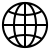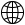# Basic Excel Functions: No More Long Division!

###### 7th August, 2023 I by WebSiteAdmin

Welcome to this week’s Tip of the Week! If you’ve missed our previous Tips of the Week, be sure to check them out here.

I have to confess something right off the bat: I never learned long division. When I was in elementary school, and then when taking standardized tests in later years, I would always use trial and error and my knowledge of multiplication, addition, and subtraction to work around my flaw. Did I make things harder on myself? Certainly. Do I regret not putting in the time to learn it? Somewhat, yes, but with my handy TI- 83, I felt unstoppable. These days, I view this lack of knowledge as a combination of me being lazy and me being efficient. Why should I learn long division when I have a calculator on my phone and my computer, and most importantly, when I have Excel.

I use Excel all the time for calculations both simple and complex and it saves me an incredible amount of time. In this post, I’m going to go over some of the simplest Excel functions (and one advanced one) that will save you time and many a headache. These functions are the ones that I use most often, and are the ones that I believe are the most relevant to the translation industry. If you disagree, or use other functions regularly, let me know!

The SUM function in Excel is an easy one to memorize and is used to add together numbers in multiple cells. An example of where I would use this function would be a project involving two separate translators, Translator A and Translator B. We owe Translator A \$45.50 and we owe Translator B \$56.79. If we had the money we owed Translator A in cell A1 and the money we owed Translator B in cell A2, we could use the SUM function to determine the total amount of money we owed the translators for this project.

=(A1+A2)

Hit enter and boom! We owe them \$102.29.

What if we have multiple rows or columns and we want to apply the same function to all of them at once? Typing out the same function over and over again sounds way too tedious for me, and luckily for us, Excel makes it easy to apply the same function to multiple cells. You can do this by selecting the bottom right corner of the cell you want to copy and dragging it across the cells to which you want to copy it. In the below screenshots you can see that I have dragged the screenshots over using my mouse.

And here is the final result!

While I will only be doing this for the addition function, you can apply this same technique to drag functions across multiple cells for any function in Excel!

2. Subtraction

What if instead, cell A2 denoted how much our client was paying us for the translation and cell A1 denoted how much we were paying the translator? This time, we’ll use a function to subtract one cell from another:

(A2-A1)

Which returns… \$11.29.

3. Multiplication

This time let’s imagine that cell A1 denotes your interpretation rate (say \$50/hr) and A2 denotes the number of hours you worked on a specific assignment (5.75 or 5 hours 45 minutes). With the multiplication function you can automatically calculate the amount that you are owed!

=(A1*A2)

4. Division

Finally, we come to my good friend division. Let’s pretend that you receive a 13,548 word translation project (cell A1) and are asked to give your client a turnaround time. You know that you can translate 2500 words/day (cell A2), how many days will it take you?

=(A1/A2)

Hit enter and you know how many days to quote, about 5 ½.

5.  Expert bonus: word counts in Excel

Say you receive a new translation in Excel. Now, if you aren’t using a word count software (and even if you are since you won’t necessarily be translating every cell), you may be asking yourself, how do I get an accurate word count? Before answering, I just want to say, I’m sorry; this function is not easy and not intuitive. I don’t have it memorized and I expect that few people do. I would definitely recommend copy/pasting this one (do you remember the shortcut keys?).

=IF(LEN(TRIM(A1))=0,0,LEN(TRIM(A1))-LEN(SUBSTITUTE(A1," ",""))+1)

Wow, that’s a lot, so I’ll try to break the process down. I won’t get too technical, but instead will explain what each part of the function is doing.

• The first thing to look at is the “A1” that appears throughout the function. This specifies the cell that is being analyzed, if you want the word count of another cell, just plug the other cell in instead (e.g. A2).
• The first part, IF(LEN(TRIM(A1))=0,0, determines if the cell is empty, and if it is, it returns “0.”
• The second part, LEN(TRIM(A1)), says that if the cell isn’t empty, count the number of characters in that cell. Think of this as value “A.” Note that this value includes spaces in the cell.
• The third part, -LEN(SUBSTITUTE(A1," ","")), removes the spaces and then counts the characters again. Think of this as value “B.” Then, the function does the calculation “A - B.”
• Finally, the “+1” adds one word to adjust for the first word.

If you’re like me, you need to see some examples before you truly understand it, so here we go.

Imagine a cell (A1) that contains the following: “My name is Nathanael Burt.” Part one would determine that the cell is not empty, so we move on. Part two determines that the number of characters is 26, so, A = 26. Part three determines that the number of characters (without spaces) is 22, so, B = 22. Then it runs A - B, which equals 4. Finally, we do the last operation, + 1, to account for the first word and end up with 5, the number of words in cell A1.

Here are the screenshots from Excel:

And once the function runs…

I hope these functions (especially the last one, if you made it through) help you see the power of Excel and the amount of time it can save you. This was definitely a long post and we went through a lot, but there was a lot to cover!

Do you have a suggestion for a Tip of the Week? Did we miss something in this post? Please let us know on social media or in the comments below!

Welcome to this week’s Tip of the Week! If you’ve missed our previous Tips of the Week, be sure to check them out here.

I have to confess something right off the bat: I never learned long division. When I was in elementary school, and then when taking standardized tests in later years, I would always use trial and error and my knowledge of multiplication, addition, and subtraction to work around my flaw. Did I make things harder on myself? Certainly. Do I regret not putting in the time to learn it? Somewhat, yes, but with my handy TI- 83, I felt unstoppable. These days, I view this lack of knowledge as a combination of me being lazy and me being efficient. Why should I learn long division when I have a calculator on my phone and my computer, and most importantly, when I have Excel.

I use Excel all the time for calculations both simple and complex and it saves me an incredible amount of time. In this post, I’m going to go over some of the simplest Excel functions (and one advanced one) that will save you time and many a headache. These functions are the ones that I use most often, and are the ones that I believe are the most relevant to the translation industry. If you disagree, or use other functions regularly, let me know!